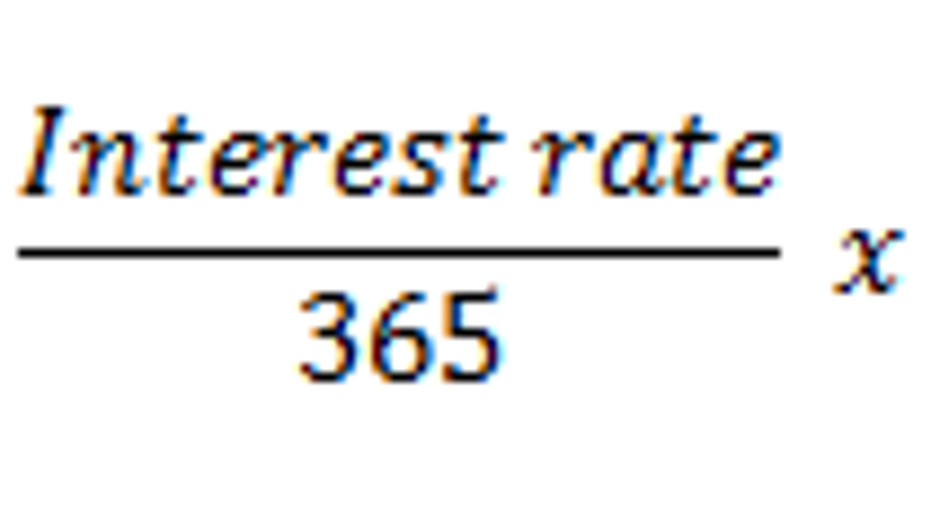# How to Calculate Simple Interest on a Per-Diem BasisSource: Flickr user Dafne Cholet.

Simple interest refers to interest that's calculated solely based on the principal, and not any interest that has already accrued. The general formula for computing simple interest is:

For example, if you borrow \$1,000 from a friend and agree to pay 6% simple interest for two years, the formula above tells you that you'll pay \$120 in total interest (\$1,000 x 0.06 x 2).

Per diem (daily) interestWhile simple interest is generally simple to calculate over the life of a loan or investment, it can also be useful to know how much interest is accruing on a daily, or "per-diem," basis.

To calculate per-diem interest, take the interest rate (be sure to express it as a decimal, so 10% becomes 0.10) and divide by 365 to determine the daily interest rate. Multiplying this amount by the principal will result in your per-diem interest. Here it is written as a mathematical formula:

As an example, let's say you want to estimate how much interest you're paying each day on your student loans. If you have \$40,000 in outstanding loans and you pay 6% simple interest, then your per diem interest is:

If you need to know the amount of interest that will accumulate over a certain number of days, then simply multiple this amount by the number of days.

Limitations of per-diem simple interestWhile simple interest is relatively easy to calculate, it's rarely used in real-world situations. Most accounts use compound interest, which means that interest accrues not only on the principal balance, but also on the interest that has already accumulated as well. As you can imagine, this is a more complicated calculation.

However, because compound interest generally isn't calculated at a more frequent rate than daily, the calculation described here can be useful to obtain a snapshot of the per-diem interest on any interest account you may have, such as a CD or mortgage. Just keep in mind that the nature of compound interest -- and a declining balance over time in the case of a loan -- will cause the per-diem interest to change as time goes on.